BASIC
ELECTRONICS COURSE
Page 1This course is an entirely new way to learn electronics. It has many features such as animations and activities that only a computer-presentation can provide. In addition, the format consists of questions and answers. Sometimes the questions are asked before the theory is provided. This is the way it happens in the real world. It is called Reverse Learning and has proven to be a much faster way to learn. There are many other features, so . . . .

.  .  . let's start.  Click your mouse on the switch:Question 1: What happens when the switch is closed?

Ans: The LED illuminates
.

Question 2: The switch completes the circuit. What does this mean?

Ans: The current (we say "the current" meaning "electricity") flows out the positive terminal of the battery, through the switch, through the LED, through the current limiting resistor and into the negative terminal of the battery

 The diagram above is called a circuit. Click on the diagram below to build the circuit. Click your mouse on each component and drag it to the correct place. Click to release and it will "fly" into position:The schematic diagram (the diagram made up of symbols) does not have to look like the layout diagram. The components may not appear in exactly the same places but the end-result is the same and both work the same. A schematic diagram generally has a defined way the components are laid out. This makes it easy for the reader to understand how the circuit works.
The schematic diagram above consists of 4 symbols (a resistor, a LED, a switch and a battery) with lines or wires to connect the components together. Here are the 4 symbols: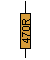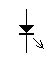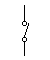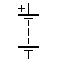There are over 100 electronic symbols in our Library of Symbols

Question 3: What do the letters LED mean?

Ans: Light Emitting Diode.The length of the leads of a component (symbol) on a circuit diagram does not indicate the actual length of the leads. In reality the cathode lead of a LED is the shorter of the two. If you cut both leads the same length, you have to refer to the small flat spot on the side of the LED to identify the cathode lead. (Or connect the LED to a battery via a resistor!)

Question 4: A Light Emitting Diode has two leads, name them.

Ans: Anode and cathode.

Question 5. How do you identify the cathode lead on an LED?

Ans: It is the shorter lead. A flat on the side of the LED also indicates the cathode lead.

Question 6: How do you connect a LED to a supply voltage?

Ans: The anode is connected to the positive of the supply and the cathode is connected to the negative via a  CURRENT LIMITING RESISTOR.

Question 7: Why do you need a current limiting resistor?

Ans: The current limiting resistor prevents too much current flowing through the LED and damaging it.

Question 8. Can the current limiting resistor be put on the anode lead?

Ans: Yes. It doesn't matter which lead has the current limiting resistor, providing it is always included.Question 9: What does the negative rail mean?

Ans: It means the negative of the battery is connected to this rail.
It can also be referred to as 0v or earth. These all mean the negative rail.

Question 10. Why is the current limiting resistor needed?

Ans: A LED is not a globe. It has no filament and it does not get hot.
It is an electronic device and is nothing like a globe at all. The only similarity is they both give off light.   Light from a LED is produced by current flowing through a crystal structure and if too much current flows, the crystal will be damaged.

Question 11. How much current does a LED need to produce light?

Ans:  It requires about 25mA to produce a very good glow. A LED will start to glow at 1mA and get brighter as the current increases.

Question 12. What colours are produced by LEDs?

Ans: A LED does not give off white light. It gives off coloured light. The most common colours are:
Red, green,
orange, yellow and blue.

Question 13. How can you make a LED give off while light?

Ans: If a red, green and blue LED are combined together and connected to a supply, the combined light output from the three LEDs will appear as a source of white light.

Question 14. If you connect a LED around the wrong way, will it work?

Ans: No. It will not illuminate. It may not be damaged but it will NOT work.Question 15. What will happen if you connect a LED to the supply rail without a current limiting resistor.

Ans: A very large current will flow through the LED and it will be instantly
damaged. This is one of the major differences between a globe and a LED.THE FLASHING LED
The Flashing LED is not a normal LED. It has a light emitting semiconductor inside the body and it also contains a microchip flasher circuit and a current limiting resistor so the LED can be connected directly to a 5v to 9v supply. It flashes about once per second. This is not the type of LED we are talking about in this discussion. The animation below shows a flashing LED connected directly to a 9v battery. Don't confuse the two. The only way to tell them apart is to connect each to a 9v battery WITH A CURRENT LIMITING RESISTOR. If one LED flashes, you can remove the resistor and it will flash. The other does not flash and is the type we are talking about in this discussion.No current-limiting resistor
is needed for a flashing LED

Now, back to the questions:

Question 16. How do you get the correct brightness from a LED?

Ans: Carefully try different values of resistance until the correct brightness is obtained. (We will go into more detail later). When we say the current limiting resistance is "too high" we mean its actual value in ohms is too high - not how high it is on the circuit diagram.  The circuit diagram is only a representation to show how the components are connected together. They are connected together with wires or leads or maybe tracks on a printed circuit board.Question 17: How does the value of the dropper resistor control the light
output from a LED?

Ans: It controls the current through the circuit.
A dropper resistor is like a narrow part in a hose - like standing on a hose.
If you stand on a hose you increase the "resistance to the flow of water."
A high value resistor reduces the flow of electricity and the LED is dull. A low value of resistance allows a high current to flow - just like removing your foot from the hose and the LED increases in brightness. A piece of wire has a low resistance and special carbon compounds have a high resistance. A resistor is made from carbon compounds and if is has a very low resistance it is said to have LOW OHMS.

Question 18. What are the values of resistance for a resistor?

Ans: Resistors can be made in any value, from zero ohms to millions of ohms. Typical values of resistance for Q 16 would be as follows: For the dull LED, the resistor would be in the range 2,000 to 5,000 ohms. For correct brightness the resistor would be about 250 to 300 ohms. To damage the LED the resistor would be less than 100 ohms. The main thing to remember is this: As the resistance of the dropper resistor DECREASES, the current flow through the circuit INCREASES. The easiest way to remember this is to use the extremes: A very high resistance is similar to an open switch and a very low resistance is similar to a piece of wire in a circuit. The open switch allows NO current to flow and the wire allows a high current to flow.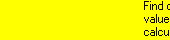In the subscription section we have pages to show you more about the resistor and a COLOUR CODE calculator for resistors. It covers the standard values for resistors.Question 19.  How does a resistor in a circuit compare to water flowing through a pipe?

Ans: A low value resistor is like a thick pipe - lots of water flows - lots of current flows. A high value resistor is like a pipe with a restriction - little water flows - only a low current will flow.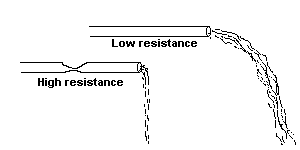Question 20: A LED is not like a globe, it doesn't get hot, it doesn't give off white light, and it doesn't require very much current. Are there an other characteristics?

Ans: Yes the voltage across a LED remains constant, even when its light output is dull or bright. This is called a "characteristic voltage drop" across the LED and is fixed for a particular colour and a particular quality of LED (some LEDs produce a high output when 25mA flows wile others give only a very dull output. The bright LEDs are called HIGH-BRIGHT and SUPER HIGH-BRIGHT. The output from a LED is getting better and better as technology and production methods improve.
Some of the early LEDs produced less than 50millicandellas for 25mA current flow. Super high-bright LEDs can emit 1,000, 2,000 and even 3,000millicandellas for 25mA current flow.

Question 21: What is 1,000millicandellas?

Ans: 1,000millicandellas is equal to 1 candella or the equivalent of one standard candle.

Question 22: What is the "characteristic voltage" for a red LED?

Ans: The characteristic voltage depends on the quality of the LED (the amount of illumination produced by the LED when 25mA flows) but for a particular LED it remains FIXED throughout its life and it is constant over the full range of illumination, from dull to bright. For the purpose of this course we will say it is 1.7v for a red LED, 2.1v for a green LED and 2.3v for a yellow LED.
We can now work out the value of the current limiting resistor (in ohms) because the equation linking the voltage of the supply rail, the current flowing in the circuit and resistance of the dropper resistor is called OHMS LAW.

Question 23: What is Ohms Law?

Ans: It links "voltage in a circuit", "current in a circuit" and the "resistance of the circuit." In other words, if a circuit has a voltage supplied to it, and the circuit has a particular resistance, a current will flow according to an equation called OHMS LAW.

When you subscribe, we have a calculator that will work out any value in an Ohm's Law equation.Question 24: We know the voltage of the supply is 9v (we are using a 9v
battery) and the voltage across the LED is 1.7v. Thus the volts across the
current limiting resistor is 7.3v. The current required by the LED is 25mA (0.025Amp).
How do you work out the value of the current limiting resistor (in ohms).

Ans: See animation below: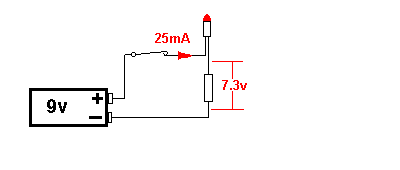Question 25: Are resistors made in every value of resistance?

Ans: No. Resistors are made is very large batches and their value cannot be made  absolutely accurately. For this reason they are measured and put into a "batch value." Typical batch values are 100 ohms, 120 ohms, 150 ohms, 180 ohms, 220 ohms, 270 ohms, 330 ohms 390 ohms and higher (as well as lower), where resistors of 90 ohms to 110 ohms are put into the 100 ohm "batch," and resistors of 111 ohm to about 135 ohms are put in the 120 ohm batch. This way all the resistors are put into a "batch" and nothing is wasted.

Question 26: What value dropper resistor should be used in the example above?

Ans: The current limiting resistor works out to be 292 ohms but a resistor of this value is not available, so the solution is to use the next higher or lower value - 270 ohms or 330 ohms.

Question 27: Can a 292 ohm resistor be obtained?

Ans: Yes. Any value of resistance can be obtained by combining resistors in parallel and/or series.